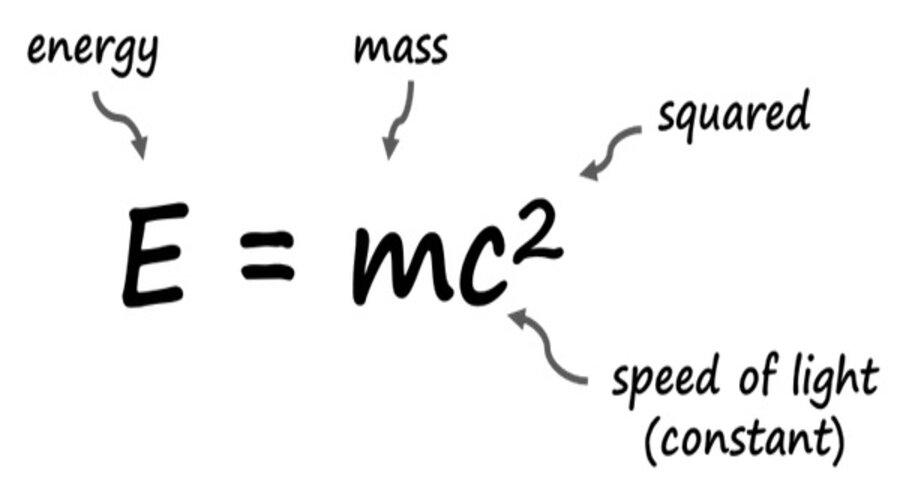# Einstein’s E=mc² ExplainedEinstein’s famous equation, E=mc², is one of the most well-known scientific formulas in the world. It states that energy (E) and mass (m) are interchangeable, and that the amount of energy contained in a given mass is equal to the mass multiplied by the speed of light squared (c²).

The equation was first introduced in 1905 by Albert Einstein in his special theory of relativity. In this theory, Einstein proposed that the laws of physics are the same for all observers moving at a constant velocity relative to one another, and that the speed of light is always constant.

One of the key implications of E=mc² is that energy and mass are not separate entities, but rather different forms of the same thing. This means that energy can be converted into mass, and vice versa. For example, when a nuclear reaction occurs, energy is released in the form of heat, light, and other forms of radiation, and a small amount of mass is converted into energy. Similarly, when a particle accelerator creates subatomic particles, energy is converted into mass.

The equation also has important implications for the field of nuclear physics. Nuclear reactions, such as those that occur in atomic bombs and nuclear power plants, involve the conversion of a small amount of mass into a large amount of energy. This is due to the fact that the speed of light squared is a very large number, making the conversion of even a tiny amount of mass into energy a significant event. This is the key principle behind the enormous energy yields of nuclear reactions, and the reason why nuclear energy is so powerful.

E=mc² also has important implications for the field of cosmology. According to the Big Bang theory, the universe began as a singularity, an infinitely dense and hot point, which expanded and cooled over time. According to the equation, this means that energy was converted into matter as the universe expanded. This process is thought to have been responsible for the formation of all the matter in the universe, including galaxies, stars, and planets.

Additionally, E=mc² has also played a critical role in the development of modern technology. For example, the development of atomic bombs and nuclear power plants were made possible by the understanding of this equation. The equation also has applications in fields such as medical imaging and radiation therapy, where the conversion of energy into mass is used to create images of the human body and to treat cancer.

In particle physics, the equation is used to calculate the mass-energy of subatomic particles such as protons and neutrons. This is important in understanding the properties and behavior of these particles, and in developing new technologies such as particle accelerators.

Einstein’s equation has also been used to calculate the energy density of the universe, which is an important parameter in understanding the overall structure and evolution of the universe. This is important in understanding the dynamics of the universe and in predicting its future behavior.

Finally, E=mc² has also been used in the study of black holes, which are extremely dense objects whose gravity is so strong that nothing, not even light, can escape from them. According to the equation, the mass of a black hole is directly proportional to its energy, making it a key tool in understanding the properties and behavior of black holes.

In summary, E=mc² is a fundamental equation in physics that describes the relationship between energy and mass. The equation states that energy and mass are interchangeable, and that the amount of energy contained in a given mass is equal to the mass multiplied by the speed of light squared. The equation has important implications for fields such as nuclear physics and cosmology, and has been instrumental in our understanding of the universe.